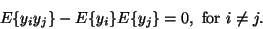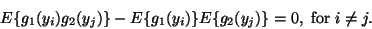Next: Definitions of linear independent Up: Independent Component Analysis Previous: Independent Component Analysis

## Statistical independence

To begin with, we shall recall some basic definitions needed. Denote by y1,y2,...,ym some random variables with joint density f(y1,...,ym). For simplicity, assume that the variables are zero-mean. The variables yi are (mutually) independent, if the density function can be factorized :

 f(y1,...,ym)=f1(y1)f2(y2)...fm(ym) (7)

where fi(yi) denotes the marginal density of yi. To distinguish this form of independence from other concepts of independence, for example, linear independence, this property is sometimes called statistical independence.

Independence must be distinguished from uncorrelatedness, which means that(8)

Independence is in general a much stronger requirement than uncorrelatedness. Indeed, if the yi are independent, one has(9)

for any2 functions g1 and g2 . This is clearly a stricter condition than the condition of uncorrelatedness. There is, however, an important special case where independence and uncorrelatedness are equivalent. This is the case when y1,...,ym have a joint Gaussian distribution (see ). Due to this property, independent component analysis is not interesting (or possible) for Gaussian variables, as will be seen below.Next: Definitions of linear independent Up: Independent Component Analysis Previous: Independent Component Analysis
Aapo Hyvarinen
1999-04-23